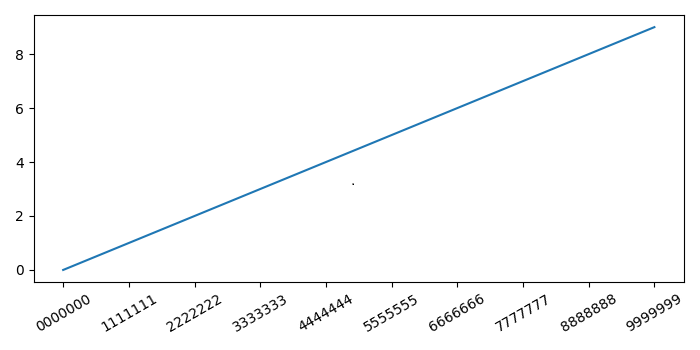# How to autosize text in matplotlib Python?

To autosize text in matplotlib, we can make a tight layout and rotate the ticks.

## Steps

• Set the figure size and adjust the padding between and around the subplots.

• Plot data points of the range of 10.

• Make a list of labels.

• Put ticks and labels on the X-axis with 30 rotation.

• To display the figure, use Show() method.

## Example

from matplotlib import pyplot as plt

plt.rcParams["figure.figsize"] = [7.00, 3.50]
plt.rcParams["figure.autolayout"] = True

plt.plot(range(10))

labels = [7 * repr(i) for i in range(10)]

plt.xticks(range(10), labels, rotation=30)

plt.show()


## Output

It will produce the following output −